## 2012/07/25

### 製造無法閱讀的程式碼

1. 做卡片
2. 轉成程式碼
3. 製造大亂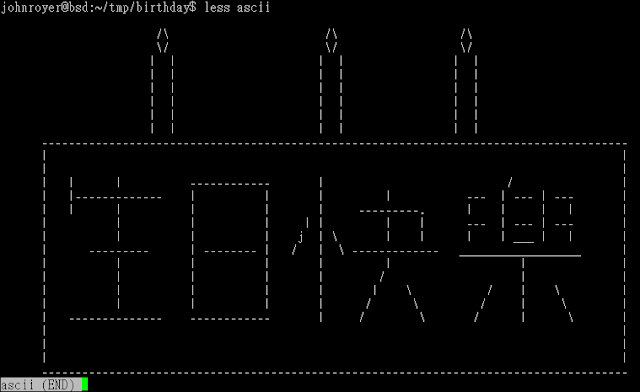``````void pt( int ascii, int count ){
int i;
for( i = 0; i < count; i++ ){
printf("%c", ascii);
}
}``````

``````#include <stdio.h>

void pt(int ascii, int count){
if( count > 0 ){
printf("%c", ascii);
pt( ascii, count-1 );
}
}

int main(){
pt(10, 1);    //   \n
pt(32, 23);   //   sapce
pt(47, 1);    //   "/"
pt(92, 1);    //   "\"
pt(32, 23);
pt(47, 1);
.....

return 0;
}``````

``pt(0xA, 10+8-17);     //   \n``

main() 中 pt() 重複次數太高，即使取代名稱以後看起來還是井然有序。所以複製出多個功能完全一模一樣的函式，並且建立另一個函式專門呼叫 printf。

``````void pt( int ascii, int count ){
if( count > 0 ){
printChar( ascii );
pt( ascii, count-1 );   // Can call pt2() and other, too
}
} ;

void pt2( int ascii, int count ){ ... } ;
void pt3( int ascii, int count ){ ... } ;
void pt4( int ascii, int count ){ ... } ;

void printChar( int ascii ){
printf("%c", ascii );
}``````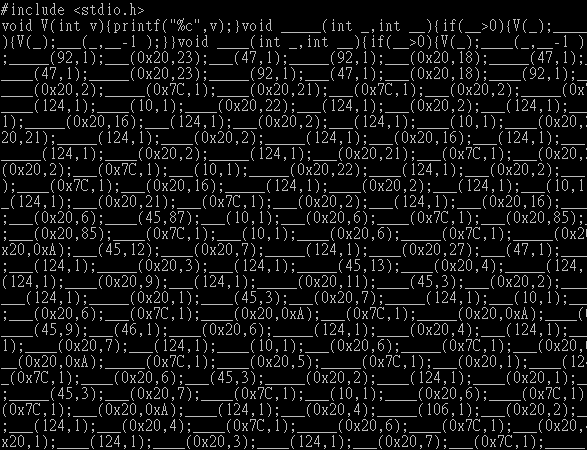gcc -S 參數可以將 C 語言轉成組合語言：
``gcc -S -o code.asm source.c``

Javascript 有現成的工具可以玩：aaencode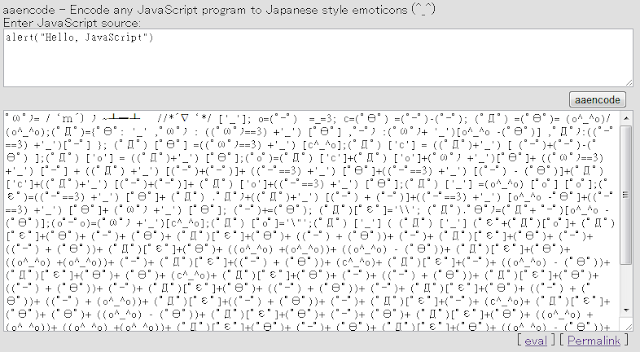#### 2 則留言:

1.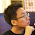2.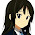樓上大神 <(_ _)>